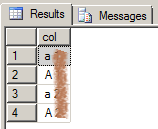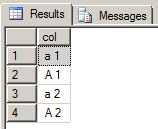# Case Sensitive collation sort order

November 23rd, 2012

A recent inquiry from one of our front line CSS engineers had me look into how case sensitive collations decide the sort order. Consider a simple question like How should the values 'a 1', 'a 2', 'A 1' and 'A 2' sort?

``````
create table [test] (
[col] varchar(10)
collate Latin1_General_CS_AS);
go

insert into [test] ([col]) values
('a 1'),
('a 2'),
('A 1'),
('A 2');
go

select [col]
from [test]
order by [col];
``````

Here are two possible outputs:

Which one is correct? A programmer will chose the first order: 'a 1', 'a 2', 'A 1', 'A 2'. Because if one would implement a string comparison routine it would compare character by character until a difference is encountered, and 'a' sorts ahead of 'A'. But this answer is wrong. The correct sort order is 'a 1', 'A 1', 'a 2', 'A 2'! And if you ran the query in SQL Server you certainly got the second output. But look again at the sort order and focus on just the first character:By default, the algorithm makes use of three fully-customizable levels. For the Latin script, these levels correspond roughly to: alphabetic ordering, diacritic ordering, case ordering

So, in a case sensitive collation, is 'a' ahead or after 'A' in the sort order? The images shows them actually interleaved, is 'a', 'A', 'a', 'A'. What’s going on? The answer is that collation sort order is a little more nuanced that just comparing characters until a difference is encountered. This is described in the Unicode Technical Standard #10: UNICODE COLLATION ALGORITHM. And yes, the same algorithm is applied for non-Unicode types (VARCHAR) too. The algorithm actually gives different weight to character differences and case differences, a difference in alphabetic order is more important than one in case order. To compare the sort order of two strings the algorithm is more like the following:

• Compare every character in case insensitive, accent insensitive manner. If a difference is found, this decides the sort order. If no difference is found, continue.
• Compare every character in case insensitive, accent sensitive manner. If a difference is found, this decides the sort order. If no difference is found, continue.
• Compare every character in case sensitive manner (we already know from the step above there is no accent difference). If a difference is found, this decides the sort order. If no difference is found the strings are equal.

Needless to say the real algorithm does not need to traverse the strings 3 times, but the logic is equivalent to above. And remember that when the strings have different lengths then the comparison expands the shorter string with spaces and compares up to the length of the longest string. Combined with the case sensitivity rules this gives to a somewhat surprising result when using an inequality in a WHERE clause:

``````
select [col]
from [test]
where [col] > 'A'
order by [col];
``````That’s right, we got back all 4 rows, including those that start with 'a'. This surprises some, but is the correct result. 'a 1' should be in the result, even though 'a' is < 'A'. If you follow the algorithm above: first we expand the shorter string with spaces, so the comparison is between 'a 1' and 'A  '. Then we do the first pass comparison, which is only alphabetic order, case insensitive and accent insensitive, character by character: 'a' and 'A' are equal, ' ' and ' ' are equal, but '1' is > ' '. The comparison stops, we found a alphabetic order difference so 'a 1' > 'A  ', the row qualifies and is included in the result. Ditto for 'a 2'.